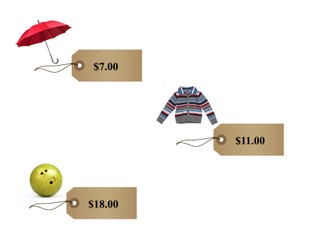Comparing and ordering numbers to 20

# Comparing and ordering numbers to 20

Comparing and ordering numbers to 20

No account needed.8,000 schools use Gynzy92,000 teachers use Gynzy1,600,000 students use Gynzy

## General

Students learn to compare numbers from 1 to 20 and are able to set them in order, they know when a number is more or less.

1.NBT.B.3

## Introduction

Hand out number cards from 1 to 20 to the students. Give the students a task, for example- everyone with a number that is more than 15 stand up. Repeat this multiple times with different numbers and alternate with giving a task with more than and less than.

## Instruction

Show the number line on the interactive whiteboard and use this to show students that one number is more than another number, and that the number values go up from left to right. Next, explain that you can set a small group of numbers in order from least to most. You do this using the number line. The number that comes first on the number line is the least or smallest number. The biggest number, or the most, is the last one on the number line. As a class, compare a few numbers and set them in correct numerical order.

Check that students understand ordering and comparing numbers to 20 by asking the following questions:
- How do you set numbers in numerical order?
- When is one number more than another number?
- How do you know which number is the least, or smallest?
- How do you know which numbers is the most, or biggest?
- What can you use to help set numbers in the correct order?

## Quiz

Students first practice recognizing most or least between two numbers. They then practice setting multiple numbers in order from least to most.

## Closing

Discuss with students that it is important to be able to compare and order numbers to 20 so that you are able to figure out what is most expensive. Check that students know that they can use the number line to help set the numbers in their correct places and to determine which number is least and which is most. Set a selection of numbers in order on the interactive whiteboard as a class. Ask students how they determine the smallest, or least number, and the most, or biggest number.

## Teaching tips

Students who have difficulty comparing and ordering numbers can be supported by using the number line. Have them point at numbers on the number line as they discuss them to help show their place. Emphasize that if a number comes first on the number line, it is smaller than the number that comes after it.

## Instruction materials

Number cards to 20

### The online teaching platform for interactive whiteboards and displays in schools

• Save time building lessons

• Manage the classroom more efficiently

• Increase student engagement